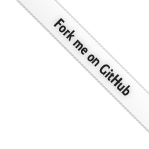# Exact Types

While binary floating-point numbers are better for computers to work with, and usually good enough for humans, sometimes they are just not appropriate. Sometimes, the numbers really must add up to the last bit, and no technical excuses are acceptable - usually when the calculations involve money.

Unfortunately, there is no dominating standard like IEEE 754 for this (The 2008 version of the standard added decimal types, which is too recent to have seen widespread adoption). Each language or platform has its own solution, sometimes multiple different ones. For details, look at the language cheat sheets.

There are at least three fundamentally different kinds of such types:

## Limited-Precision Decimal

Basically the same as a IEEE 754 binary floating-point, except that the exponent is interpreted as base 10. As a result, there are no unexpected rounding errors. Also, this kind of format is relatively compact and fast, but usually slower than binary formats.

## Arbitrary-Precision Decimal

Sometimes called “bignum”, this is similar to a limited-precision type, but has the ability to increase the length of the significand (possibly also the exponent) as required. The downside is that there is some basic overhead (memory and speed) to support this flexibility, and that the longer the significand gets, the more memory is needed and the slower all calculations become.

It can be very tempting to say “My calculation is important, so I need as much precision as possible”, but in practice the actual importance of precision at the 10,000th decimal digit quickly pales in comparison with the performance penalty required to support it.

## Symbolic calculations

The “holy grail” of exact calculations. Achieved by writing a program that actually knows all the rules of math and represents data as symbols rather than imprecise, rounded numbers. For example:

• 1/3 is actually a fraction “one divided by three”
• The square root of 2 is really the number that, multiplied by itself, is exactly 2
• Even transcendental numbers like e and π are known, together with their properties, so that e is exactly equal to -1.

However, these symbolic math systems are complex, slow and require significant mathematical knowledge to use. They are invaluable tools for mathematicians, but not appropriate for most everyday programming tasks. And even many mathematicians work on problems where imprecise, numerical solutions are better because no symbolic solution is known.